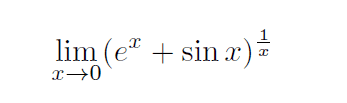# Small limit problem

Stuck on really starting this onerelevant info: Same chapter as L’Hospital’s Rule.

Any ideas?

$$ln(y)= ln((e^x+ sin(x))^{1/x})= \frac{ln(e^x+ sin(x)}{x}$$